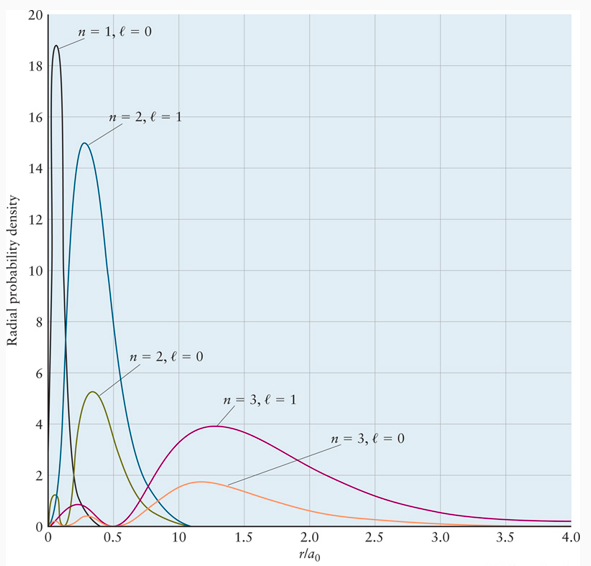# In the following plot of the radial distribution function, x-axis represents the distance from the nucleus and the y-axis represent the probability of finding an the electron. Several different orbitals are plotted and labeled using quantum numbers. In the plot below, click on the region of the function that represents the 2s orbital penetrating the 1s core electrons.